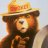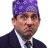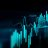# Convert Range indicator from PineScript to thinkscript?

J

#### javin82

##### New member
My previous post got deleted because it lacked sufficient information so I will try posting this again with a little more detail.

I need help converting a pine script indicator into a think script study for Thinkorswim.
The name of the indicator is called Range.
It is a reversal indicator and plots long and short signals
If you can help me with this, please let me know. Thank you!

PineScript code below:
Code:
``````//////////////
//@version=3
study("Range fv2",overlay=true)
showTrendLine = input(false, title="Show Trend Line")
colorBars = input(true, title="Color Bars?")
filterTrend = input(true, title="Filter Trend?")

//Source
src = close

//Sampling Period
per = input(defval=25, minval=1, title="Sampling Period")

//Range Multiplier
mult = input(defval=2, minval=0, title="Range Multiplier")

//-----------------------------------------------------------------------------------------------------------------------------------------------------------------
//Definitions
//-----------------------------------------------------------------------------------------------------------------------------------------------------------------

//Smooth Average Range
smoothrng(x, t, m)=>
wper      = (t/3) - 1
avrng     = sma(abs(x - x), t)
smoothrng = sma(avrng, wper)*m
smoothrng
smrng = smoothrng(src, per, mult)

//Range Filter
rngfilt(x, r)=>
rngfilt  = x
rngfilt := x > nz(rngfilt) ? ((x - r) < nz(rngfilt) ? nz(rngfilt) : (x - r)) : ((x + r) > nz(rngfilt) ? nz(rngfilt) : (x + r))
rngfilt
filt = rngfilt(src, smrng)

//Filter Direction
upward   = 0.0
upward  := filt > filt ? nz(upward) + 1 : filt < filt ? 0 : nz(upward)
downward = 0.0
downward := filt < filt ? nz(downward) + 1 : filt > filt ? 0 : nz(downward)

//Target Bands
hband = filt + smrng
lband = filt - smrng

//Colors
filtcolor = upward > 0 ? lime : downward > 0 ? red : orange
barcolor  = (src > filt) and (src > src) and (upward > 0) ? lime : (src > filt) and (src < src) and (upward > 0) ? green : (src < filt) and (src < src) and (downward > 0) ? red : (src < filt) and (src > src) and (downward > 0) ? maroon : orange
barcolor(colorBars ? barcolor : na)

//-----------------------------------------------------------------------------------------------------------------------------------------------------------------
//Plots
//-----------------------------------------------------------------------------------------------------------------------------------------------------------------

//Range Filter
if filterTrend == true
showTrendLine := false
filtplot = plot(filterTrend ? filt : na, color=filtcolor, linewidth=4, title="Range Filter")
//
Factor=input(3, minval=1,maxval = 100)
Pd=input(3, minval=1,maxval = 100)

Up=hl2-(Factor*atr(Pd))
Dn=hl2+(Factor*atr(Pd))
TrendUp = 0.0
TrendDown = 0.0
linecolor = na
up = na
down = na
Tsl = 0.0
Trend = 0.0
TrendUp := close>TrendUp? max(Up,TrendUp) : Up
TrendDown := close<TrendDown? min(Dn,TrendDown) : Dn
Trend := close > TrendDown ? 1: close< TrendUp? -1: nz(Trend,1)
Tsl := Trend==1? TrendUp: TrendDown
linecolor := Trend == 1 ? green : red
plot(showTrendLine ? Tsl : na, color = linecolor , style = line , linewidth = 3,title = "SuperTrend")

// Strategy

longCond = na
shortCond = na
longCond :=crossunder(Tsl,filt)
shortCond :=crossover(Tsl,filt)

// Count your long short conditions for more control with Pyramiding

sectionLongs = 0
sectionLongs := nz(sectionLongs)
sectionShorts = 0
sectionShorts := nz(sectionShorts)

if longCond
sectionLongs := sectionLongs + 1
sectionShorts := 0

if shortCond
sectionLongs := 0
sectionShorts := sectionShorts + 1

// Pyramiding

pyrl = 1

// These check to see your signal and cross references it against the pyramiding settings above

longCondition = longCond and sectionLongs <= pyrl
shortCondition = shortCond and sectionShorts <= pyrl

// Get the price of the last opened long or short

last_open_longCondition = na
last_open_shortCondition = na
last_open_longCondition := longCondition ? open : nz(last_open_longCondition)
last_open_shortCondition := shortCondition ? open : nz(last_open_shortCondition)

// Check if your last postion was a long or a short

last_longCondition = na
last_shortCondition = na
last_longCondition := longCondition ? time : nz(last_longCondition)
last_shortCondition := shortCondition ? time : nz(last_shortCondition)

in_longCondition = last_longCondition > last_shortCondition
in_shortCondition = last_shortCondition > last_longCondition

// Take profit

isTPl = input(true, "Take Profit Long")
isTPs = input(true, "Take Profit Short")
tp = input(5, "Take Profit ", type=float)
long_tp = isTPl and crossover(high, (1+(tp/100))*last_open_longCondition) and longCondition == 0 and in_longCondition  == 1
short_tp = isTPs and crossunder(low, (1-(tp/100))*last_open_shortCondition) and shortCondition == 0 and in_shortCondition == 1

// Create a single close for all the different closing conditions.

long_close = long_tp  ? 1 : 0
short_close = short_tp ? 1 : 0

// Get the time of the last close

last_long_close = na
last_short_close = na
last_long_close := long_close ? time : nz(last_long_close)
last_short_close := short_close ? time : nz(last_short_close)

bton(b) => b ? 1 : 0
plotshape(longCondition , title="Long", color=green, textcolor=green, transp=0,
style=shape.triangleup, location=location.belowbar, size=size.small,text="LONG",offset=0)

plotshape(shortCondition, title="Short", color=red, textcolor=red, transp=0,
style=shape.triangledown, location=location.abovebar, size=size.small,text="SHORT",offset=0)

//plotshape(longCondition, title = "BUY Signal", text = "Buy", style=shape.triangleup, location=location.belowbar, color = blue, editable = false, transp = 0)
//plotshape(shortCondition, title = "SELL Signal", text = "Sell", style=shape.triangledown, location=location.abovebar, color = black, editable = false, transp = 0)

plotshape(long_tp and last_longCondition > nz(last_long_close), text ="TP", title="Take Profit Long", style=shape.triangledown,
location=location.abovebar, color = red, editable = false, transp = 0)
plotshape(short_tp and last_shortCondition > nz(last_short_close) , text ="TP", title="Take Profit Short", style=shape.triangleup,
location=location.belowbar, color = lime, editable = false, transp = 0)

alertcondition(bton(long_tp and last_longCondition > nz(last_long_close)), title="Take Profit Long")
alertcondition(bton(short_tp and last_shortCondition > nz(last_short_close)), title="Take Profit Short")``````
Any help I can get with this will be greatly appreciated! Thank you!

J

#### javin82

##### New member
bump. I really need some help. Anyone interested in helping me? Any reply would be appreciated.How do I convert this ATR% chart study into a scanner watchlist column? Questions 4Convert encoded Mt4 to Thinkscript Questions 2Convert Chande Kroll Stop to ThinkorSwim Questions 2